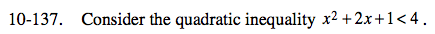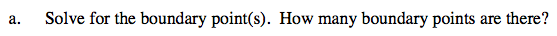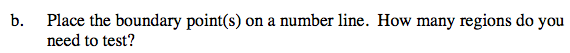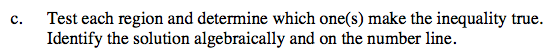### Home > CCA > Chapter 10 > Lesson 10.3.3 > Problem10-137

10-137.
1. Consider the quadratic inequality x2 + 2x + 1 < 4. Homework Help ✎

1. Solve for the boundary point(s). How many boundary points are there?

2. Place the boundary point(s) on a number line. How many regions do you need to test?

3. Test each region and determine which one(s) make the inequality true. Identify the solution algebraically and on the number line.Rearrange the inequality so that you can factor it.
How many solutions do you get when you do this?

There are 2 boundary points. x = 1 and x = −3.If there are 2 boundary points, how many regions are there surrounding those two points on a number line?Substitute a point from each one of these regions into the inequalities.
Does that point make the inequality true? If not, then it is not included.

−3 < x < 1.
Make sure to draw this on a number line.